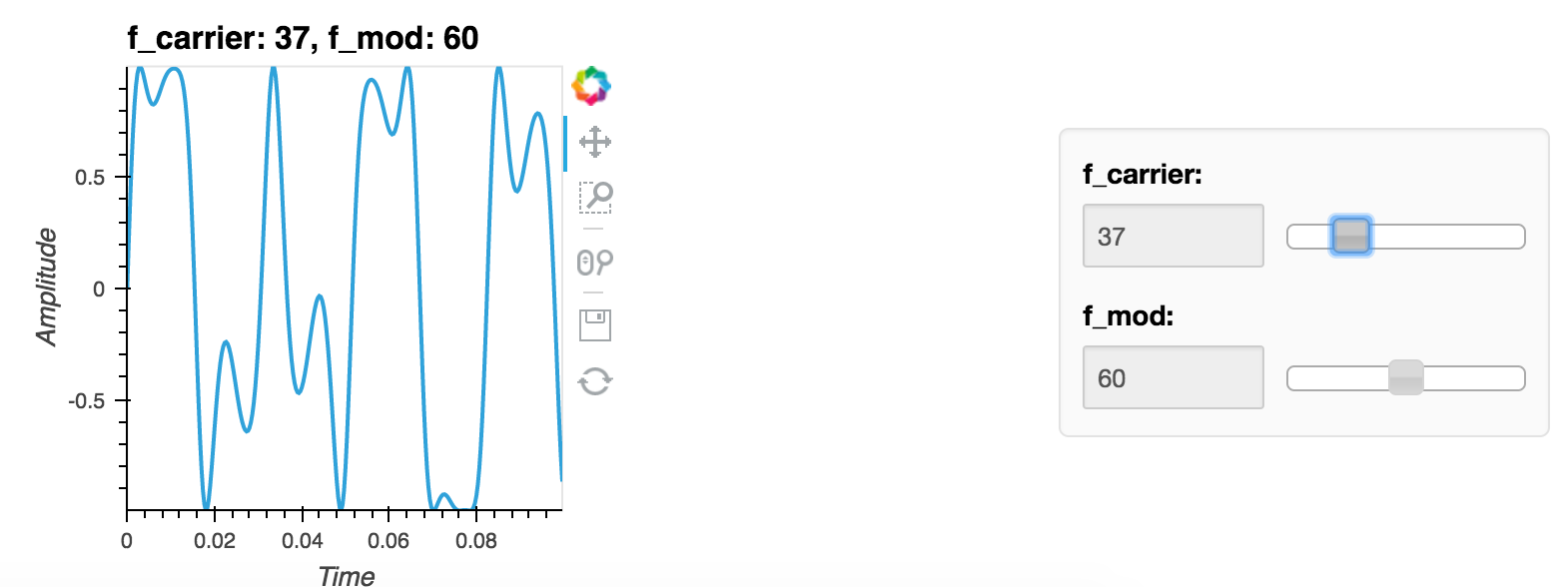# pyviz/holoviews

Have a question about this project? Sign up for a free GitHub account to open an issue and contact its maintainers and the community.

By clicking “Sign up for GitHub”, you agree to our terms of service and privacy statement. We’ll occasionally send you account related emails.

# redim.step only works for float dimensions, not integers #1707

Closed
opened this Issue Jul 10, 2017 · 3 comments

Projects
None yet
Contributor

### jbednar commented Jul 10, 2017

 ``````import numpy as np import holoviews as hv hv.extension('bokeh') def fm_modulation(f_carrier=110, f_mod=110, mod_index=1, length=0.1, sampleRate=3000): x = np.arange(0, length, 1.0/sampleRate) y = np.sin(2*np.pi*f_carrier*x + mod_index*np.sin(2*np.pi*f_mod*x)) return hv.Curve((x, y), kdims=['Time'], vdims=['Amplitude']) dmap = hv.DynamicMap(fm_modulation, kdims=['f_carrier', 'f_mod']) dmap = dmap.redim.range(f_carrier=((10, 110)), f_mod=(10.0, 110.0)) dmap = dmap.redim.step(f_carrier=10).redim.step(f_mod=10) dmap ``````Contributor

### jlstevens commented Jul 11, 2017

 I didn't realize it in the tutorial but the issue was the declaration of ranges as ints, not floats. What is wrong here is that using a float step value should be respected even if the the ranges were set using integers.
Contributor Author

### jbednar commented Jul 11, 2017

 We may be talking about different things here, because in the code above I'm telling both dims to have an integer step of 10, but f_carrier is ignoring the step, even though it's defined to have an integer range, whereas f_mod is respecting the step. So this is not an example of using a float step value, right?
Contributor

### jlstevens commented Jul 11, 2017

 Seems like there may be two related problems here.

###philippjfr referenced this issue Mar 3, 2018

Merged

#### Fixes for widget step and sorting #2390

2 of 2 tasks complete

###jlstevens closed this in #2390 Mar 4, 2018

to join this conversation on GitHub. Already have an account? Sign in to comment
You can’t perform that action at this time.